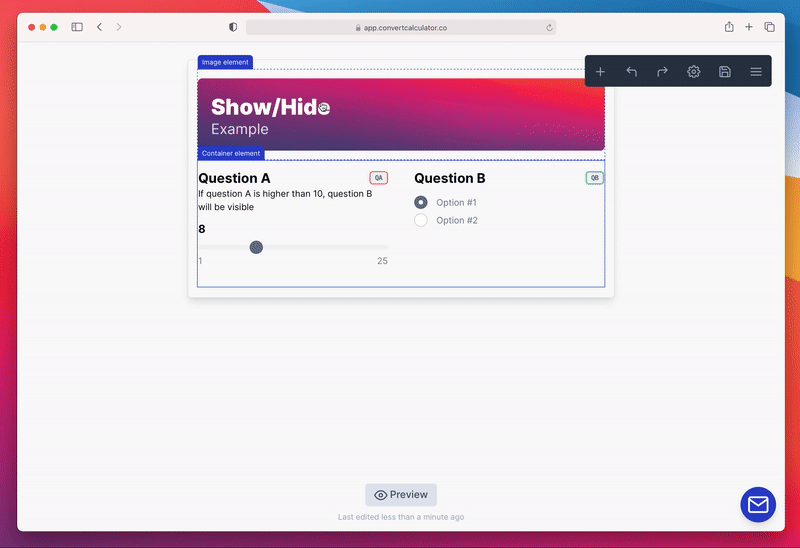# Show/hide logic on form items

Learn how to conditionally show or hide form items with an equation

We've created show/hide logic to give you the superpower to create a conditional form that only shows the relevant items.

If you want to control the visibility of an item, make sure you are in the edit screen of that item and toggle the option "Show/hide logic" on. Now, a new formula field is visible called "Visibility equation" that you can use to control the visibility.

When the result of the visibility equation is higher than `0` or `TRUE`, the item is visible. If it's `0` or `FALSE`, it's hidden.# Examples

Our conditional forms with show/hide-logic are best explained by some examples:

## If QA is higher than 10, hide QB

Visibility equation of QB:

`FS`IF(QA > 10, FALSE, TRUE)``
or
`FS`QA > 10``

## If VA is smaller than 10 or higher than 100, show QA

Visibility equation of QA:

`FS`IF(OR(VA < 10, VA > 100, TRUE, FALSE))``

## If QA + QB is 110, show QC, otherwise show QD

Visibility equation of QC:

`FS`IF(QA + QB = 110, TRUE, FALSE)``
or
`FS`QA + QB = 110``
Visibility equation of QD:
`FS`IF(QA + QB = 110, FALSE, TRUE)``

## Related articles